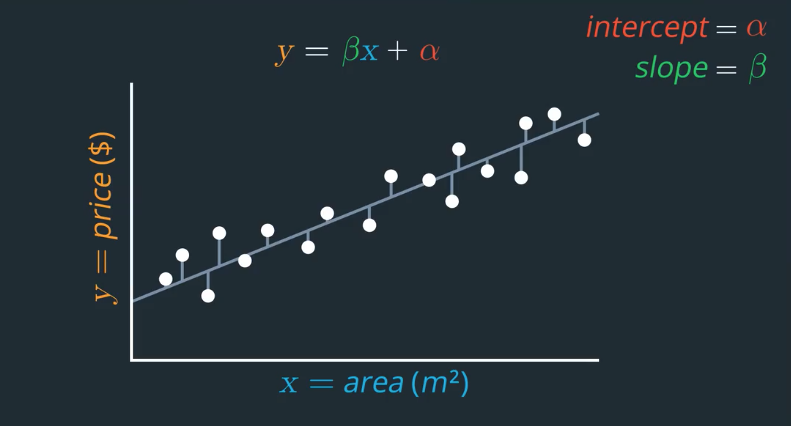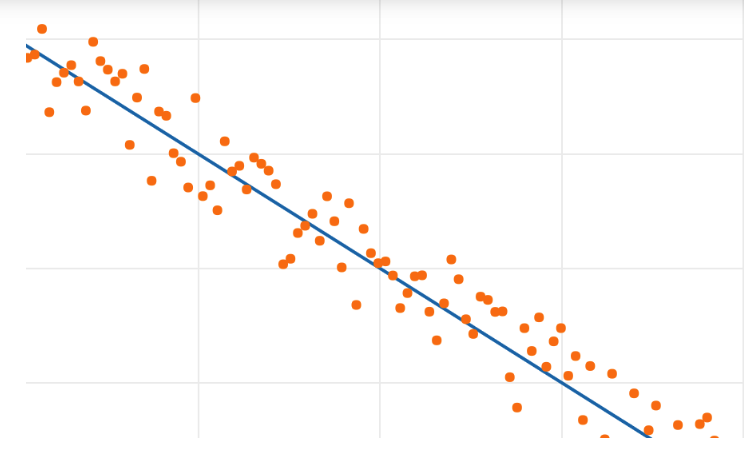# AI For Trading: Linear Regression (19)

we'll cover the basics of regression as this forms the basis for several models that are used to analyze stock returns over time. If we want to estimate the price of a house, we may assume that home buyers are willing to pay more for a bigger house. all other things being equal.### Breusch-Pagan Test for Heteroscedasticity (revisited)

Now that we’ve covered regression, let’s look at the Breusch Pagan test in more depth. The Breusch-Pagan test is one of many tests for homoscedasticity/heteroscedasticity.

It takes the residuals from a regression, and checks if they are dependent upon the independent variables that we fed into the regression. (Note that we’ll explain residuals in a few videos within this lesson, so feel free to jump back here after you watch the video “Linear Regression”).

The test does this by performing a second regression of the residuals against the independent variables, and checking if the coefficients from that second regression are statistically significant (non-zero).

If the coefficients of this second regression are significant, then the residuals depend upon the independent variables.

If the residuals depend upon the independent variables, then it means that the variance of the data depends on the independent variables. In other words, the data is likely heteroscedastic. So if the p-value of the Breusch-Pagan test is ≤ 0.05, we can assume with a 95% confidence that the distribution is heteroscedastic (not homoscedastic).

### Breusch-Pagan Test in Python

In Python, we can use the statsmodels.stats.diagnostic.het_breuschpagan(resid, exog_het) function to test for heteroscedasticity. We input the residuals from the regression of the dependent variable against the independent variables. We also input the independent variables that may affect the variance of the data. The function outputs a p-value.

### quizeIs the coefficient in the regression line above positive, negative, or zero?

A : Positive
B: Negative
C: Zero